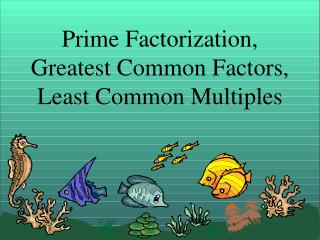# Prime Factorization, Greatest Common Factors, Least Common Multiples - PowerPoint PPT PresentationDownload PresentationPrime Factorization, Greatest Common Factors, Least Common Multiples

Prime Factorization, Greatest Common Factors, Least Common MultiplesDownload Presentation## Prime Factorization, Greatest Common Factors, Least Common Multiples

- - - - - - - - - - - - - - - - - - - - - - - - - - - E N D - - - - - - - - - - - - - - - - - - - - - - - - - - -
##### Presentation Transcript

1. What numbers can be multiplied together to get 24? The number 24 is called the product because it is the result of multiplying two or more numbers together. 1 x 24 2 x 12 3 x 8 The numbers 1, 2, 3, 4, 6, 8, 12, and 24 are called factors. These are the numbers being multiplied together. 4 x 6 What are the factors of seven? 1 x 7 Composite numbers – are numbers which have two or more factors like the number 24. Prime numbers – are numbers having exactly two factors: one and itself. Seven is a prime number.

2. Prime Factorization Factor 56 using only prime factors. To do this, we can make a factor tree as follows. 56 28 is composite so we can factor it further. 2 is prime. 2 • 28 14 is composite, so we can factor it further. 2 is prime. 2 • 14 2 is prime. 7 is prime. • 2 7 The prime factorization of 56 is 2 • 2 • 2 • 7 or 23 • 7.

3. Prime Factorization or We can make a factor tree using different starting factors. 56 14 is composite so we can factor it further. 4 is composite so we can factor it further. 4 • 14 • 2 • 2 2 7 2 is prime. 7 is prime. The prime factorization of 56 is 2 • 2 • 2 • 7 or 23 • 7. The prime factorization of every number is unique. No matter what factors you choose, the prime factorization will still be the same for that number.

4. Greatest Common Factor (GCF) • The greatest common factor is the largest factor that two numbers share. • Let’s find the GCF of 12 and 42. First, we need to make a list of factors for each number.

5. 12 42 1 x 12 1 x 42 Factors of 12: 1, 2, 3, 4, 6,12 2 x 21 2 x 6 3 x 14 3 x 4 4 x ?? 4 x 3 Factors of 42: 1, 2, 3, 6, 7, 14, 21, 42 5 x ?? 6 x 7 7 x 6 Common Factors: 1, 2, 3, 6 Greatest Common Factor: 6

6. What is the GCF of 18 and 27? 18 27 Factors of 18: 1, 2, 3, 6, 9, 18 1 x 18 1 x 27 2 x ? 2 x 9 3 x 9 3 x 6 Factors of 27: 1, 3, 9, 27 4 x ? 5 x ? 4 x ? 6 x ? 5 x ? 7 x ? Common Factors: 1, 3, 9 8 x ? 6 x 3 9 x 3 GCF: 9

7. What is the GCF of 48 and 60? Factors of 48: 1, 2, 3, 4, 6, 8, 12, 16, 24, 48 48 60 1 x 48 1 x 60 2 x 30 2 x 24 3 x 20 3 x 16 4 x 15 Factors of 60: 1, 2, 3, 4, 5, 6, 10, 12, 15, 20, 30, 60 4 x 12 5 x 12 6 x 8 6 x 10 Common Factors: 1, 2, 3, 4, 6, 12 GCF: 12

8. Least Common Multiple (LCM) • The least common multiple is the smallest number that is common between two lists of multiples.

9. EXAMPLE: Find the LCM of 12 and 18 • The multiples of 12: • 12 x 1 = 12 • 12 x 2 =24 • 12 x 3 = 36 • 12 x 4 = 48 • 12 x 5 =60 • The multiples of 18: • 18 x 1 = 18 • 18 x 2 = 36 • 18 x 3 = 54 • 18 x 4 = 72 • 18 x 5 = 90

10. 12, 24, 36, 48, 60 18, 36, 54, 72, 90 The first number you see in both lists is 36. The least common multiple of 12 and 18 is 36.

11. Example 2: Find the LCM of 9 and 10 9, 18, 27, 36, 45, 54, 63, 72 81, 90, 99 10, 20, 30, 40, 50, 60, 70, 80 90, 100, 110 If you don’t see a common multiple, make each list go further. The LCM of 9 and 10 is 90

12. Example 3:Find the LCM of 4 and 12 4, 8, 12, 16 12, 24, 36 Answer: 12

13. Example 4:Find the LCM of 5 and 8 5, 10, 15, 20, 25, 30 35, 40 8, 16, 24, 32, 40, 48 Answer: 40

14. Example 5:Find the LCM of 6 and 20 6, 12, 18, 24, 30, 36 42, 48, 54, 60 20, 40, 60, 80, 100, 120 Answer: 60